# A bar chart 5 ways in ggplot2

Andy, Tom, and ggplot2 walk into a bar…

Thomas Mock https://twitter.com/thomas_mock
08-01-2020

## CreditAndy Kirk put together Five Ways to present bar charts as part of his `Five ways to...` series back in 2019. The plots below are his original ideas, just recreated in `ggplot2`.

I originally recreated his plots in `ggplot2` and published them as a gist and on Twitter in July 2019, stumbled upon it again recently, and thought why not capture it as a proper blog-post!

Additionally, when I originally made these remakes, `ggplot2` required `coord_flip()` whereas the most recent version of `ggplot2` allows you to natively create horizontal bar charts! I’ve thus changed a little bit of the code from the original gist to reflect the new options in `ggplot2`.

Again thank you to Andy Kirk for the prompt! Make sure to check out his blog in general for all sorts of great data viz tips.

## Source Data

The data comes from Wikipedia, specifically a list of the most streamed songs on Spotify. We can scrape the table into R w/ `rvest`.

``````library(rvest)
library(tidyverse)
``````

Now that we have the libraries loaded, let’s read in the data, pull in the top 100, and add some new columns to use across our charts.

``````url <- "https://en.wikipedia.org/wiki/List_of_most-streamed_songs_on_Spotify"

df <- url %>%
read_html() %>%
html_table(fill = TRUE) %>%
.[] %>%
set_names(nm = c("rank", "song_name", "artist", "album", "streams", "date_published")) %>%
slice(1:100) %>%
mutate(num_rank = parse_number(rank),
streams_comma = streams,
streams = parse_number(streams)/1000,
streams_text = if_else(
num_rank == 1,
paste(round(streams, digits = 2), "billion streams"),
as.character(round(streams, digits = 2))
),
lab_text = glue::glue("{rank}. {song_name} by {artist}"),
) %>%
as_tibble()

df %>% glimpse()
``````
``````Rows: 100
Columns: 10
\$ rank           <chr> "1", "2", "3", "4", "5", "6", "7", "8", "9",…
\$ song_name      <chr> "\"Shape of You\"", "\"Rockstar\"", "\"Dance…
\$ artist         <chr> "Ed Sheeran", "Post Malone featuring 21 Sava…
\$ album          <chr> "÷", "Beerbongs & Bentleys", "The Kids Are C…
\$ streams        <dbl> 2.654, 2.064, 1.963, 1.944, 1.897, 1.732, 1.…
\$ date_published <chr> "6 January 2017", "15 September 2017", "10 M…
\$ num_rank       <dbl> 1, 2, 3, 4, 5, 6, 7, 8, 9, 10, 11, 12, 13, 1…
\$ streams_comma  <chr> "2,654", "2,064", "1,963", "1,944", "1,897",…
\$ streams_text   <chr> "2.65 billion streams", "2.06", "1.96", "1.9…
\$ lab_text       <glue> "1. \"Shape of You\" by Ed Sheeran", "2. \"…``````

Data is ready to go!

# Chart 1: Font-height bars

``````font_height_bars <- df %>%
filter(num_rank <=10) %>%
ggplot(aes(y = fct_reorder(lab_text, streams), x = streams)) +
geom_col(fill = "#7dc8c4", width = 0.3) +
theme(text = element_text(family = "Nunito Bold", face = "bold", size = 14),
axis.text = element_text(face = "bold"),
axis.ticks = element_blank(),
panel.grid.minor = element_blank(),
panel.grid.major.y = element_blank(),
panel.grid.major.x = element_line(color = "lightgrey")) +
labs(x = "\nNumber of streams in billions",
y = "") +
scale_x_continuous(limits = c(0,2.700), expand = c(0, 0),
breaks = scales::breaks_pretty(n = 10)
)

ggsave("font_height_bars.png", font_height_bars, dpi = 300,
height = 6, width = 14, units = "in")
``````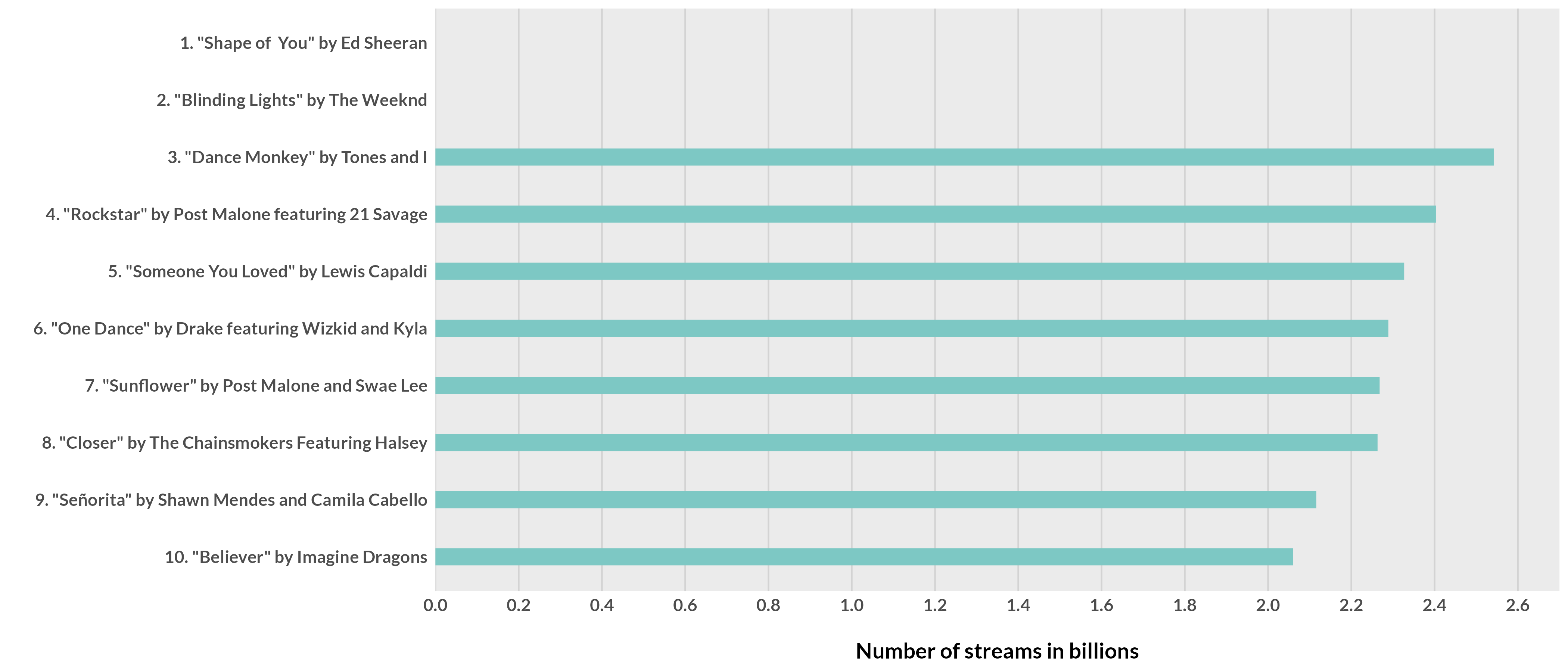# Chart 2: Bars with invisible gridlines

``````invis_gridline <- df %>%
filter(num_rank <=10) %>%
ggplot(aes(x = streams, y = fct_reorder(lab_text, streams))) +
geom_col(fill = "#3686d3", width = .9) +
geom_vline(data = data.frame(x = seq(0, 2.6, .2)),
aes(xintercept = x), color = "white", size = 0.5) +
theme_minimal() +
theme(text = element_text(family = "Nunito Bold", face = "bold", size = 14),
axis.text = element_text(face = "bold"),
axis.ticks = element_blank(),
panel.grid = element_blank()) +
labs(x = "\nNumber of streams in billions",
y = "") +
scale_x_continuous(limits = c(0,2.7), expand = c(0, 0),
breaks = scales::breaks_pretty(n = 10))

ggsave("invis_gridline.png", invis_gridline, dpi = 300,
height = 6, width = 14, units = "in")
``````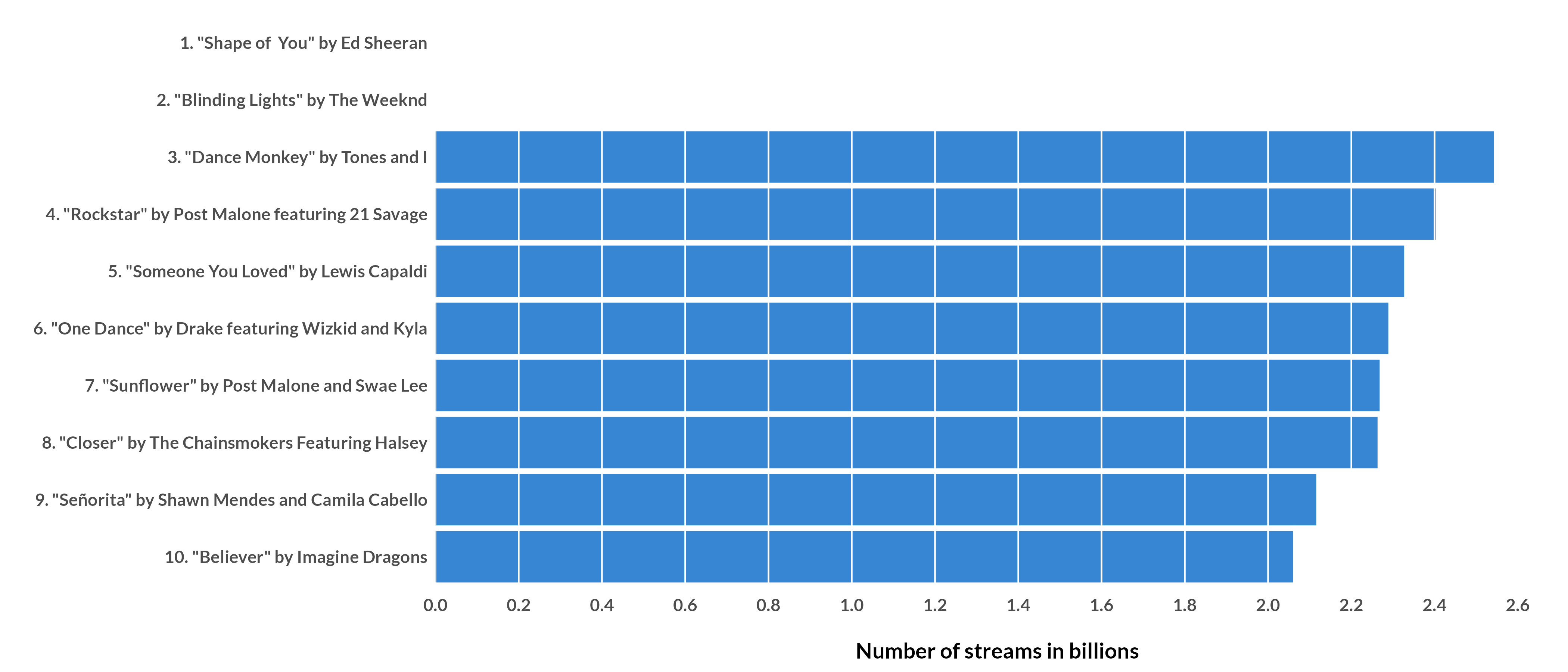# Chart 3: Direct labels

``````direct_label <- df %>%
filter(num_rank <=10) %>%
ggplot(aes(x = streams, y = fct_reorder(lab_text, streams))) +
geom_col(fill = "#303844", width = .9) +
geom_text(aes(y = fct_reorder(lab_text, streams), x = streams, label = streams_text),
color = "white", hjust = 1, fontface = "bold", position = position_nudge(x = -.020)) +
theme_minimal() +
theme(text = element_text(family = "Nunito Bold", face = "bold", size = 16),
axis.text = element_text(face = "bold"),
axis.text.x = element_blank(),
axis.ticks = element_blank(),
panel.grid = element_blank()) +
labs(y = "",
x = "") +
scale_x_continuous(limits = c(0,2.7), expand = c(0, 0),
breaks = scales::breaks_pretty(n = 10))

ggsave("direct_label.png", direct_label, dpi = 300,
height = 6, width = 14, units = "in")
``````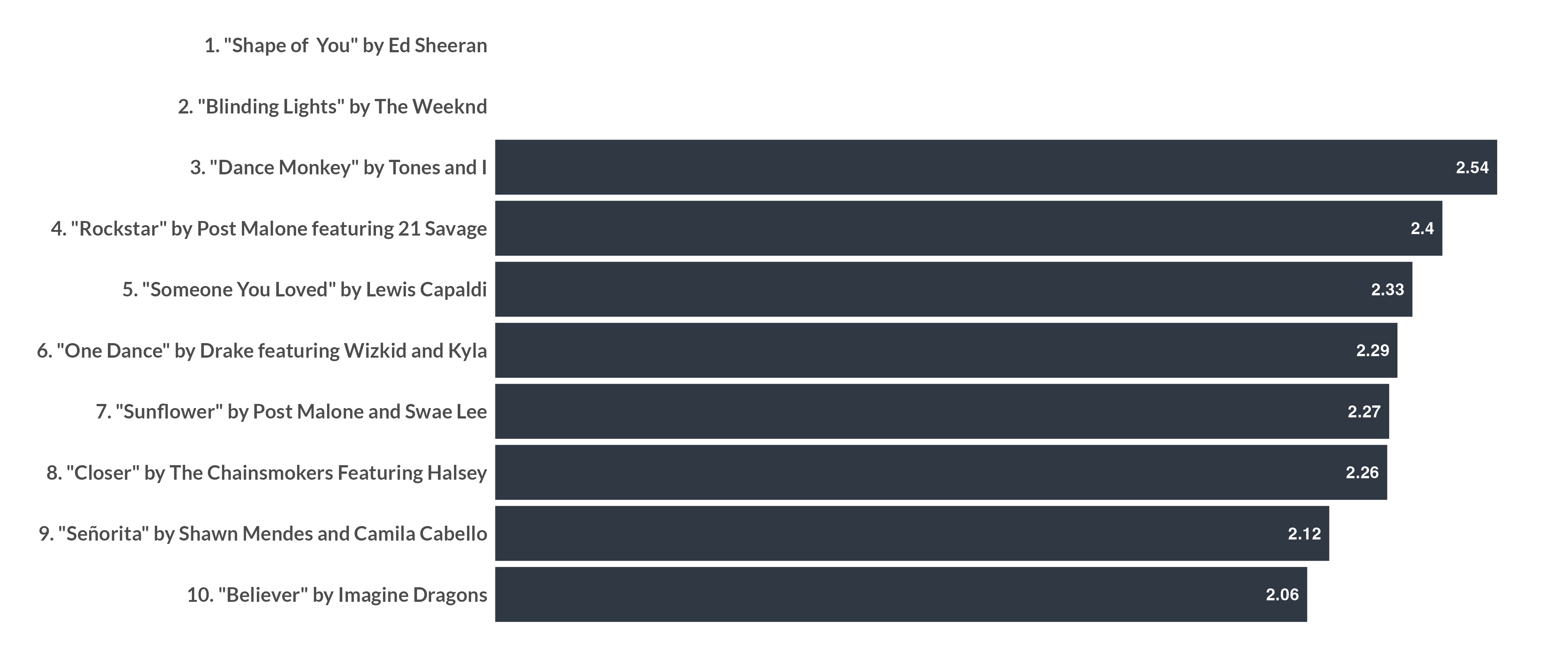# Chart 4: Labels above

``````label_above <- df %>%
filter(num_rank <=10) %>%
ggplot(aes(x = streams, y = fct_reorder(lab_text, streams))) +
geom_col(fill = "#c2545b", width = .2) +
geom_text(aes(x = 0, y = fct_reorder(lab_text, streams),  label = lab_text),
color = "black", hjust = 0, position = position_nudge(y = 0.3),
fontface = "bold", family = "Nunito Bold", size = 4) +
geom_text(aes(x = streams, y = fct_reorder(lab_text, streams), label = streams_text),
color = "#cf7a7f", hjust = 1, position = position_nudge(x = -.02, y = 0.3),
fontface = "bold", family = "Nunito Bold", size = 4) +
theme_minimal() +
theme(text = element_text(family = "Nunito Bold", face = "bold", size = 14),
axis.text = element_blank(),
axis.text.x = element_blank(),
axis.ticks = element_blank(),
panel.grid = element_blank()) +
labs(y = "",
x = "") +
scale_x_continuous(limits = c(0,2.7), expand = c(0, 0),
breaks = scales::breaks_pretty(n = 10))

ggsave("label_above.png", label_above, dpi = 300,
height = 6, width = 14, units = "in")
``````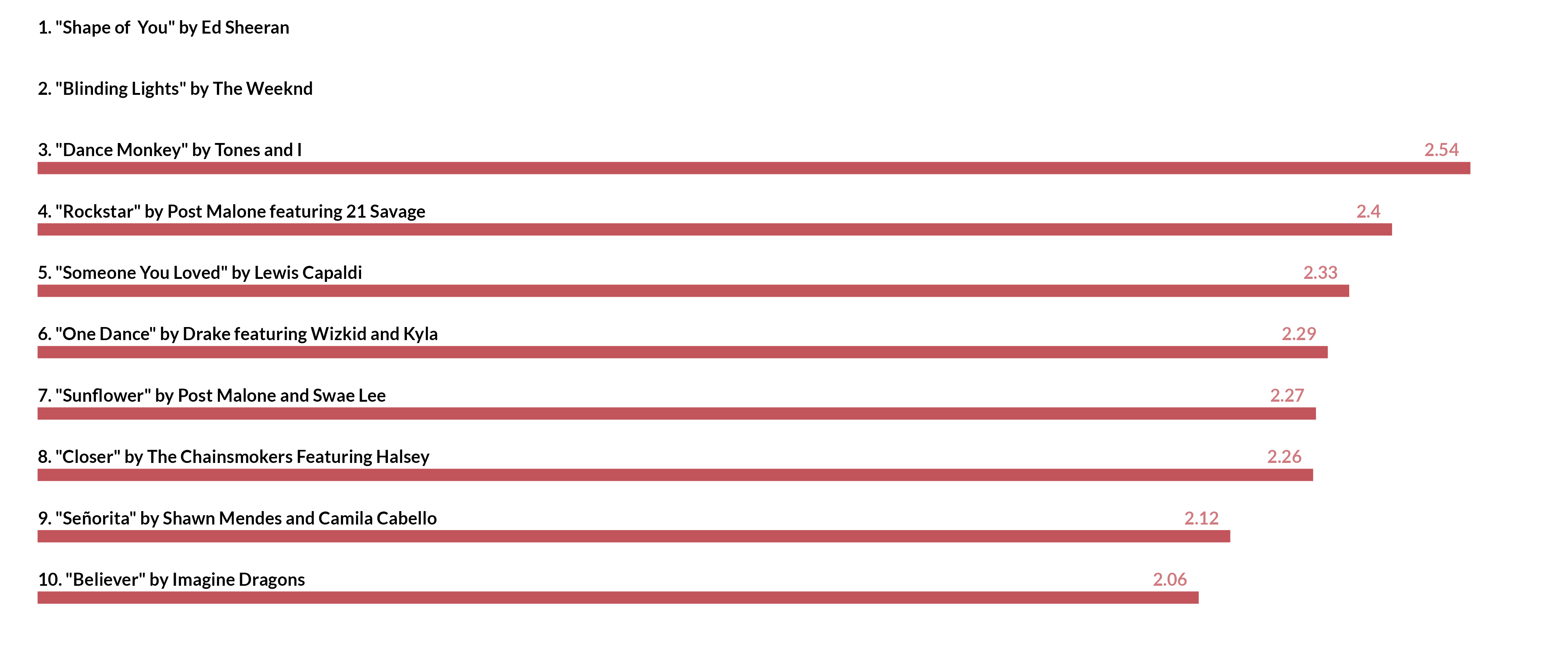# Chart 5: Lollipop

``````lollipop_bar <- df %>%
filter(num_rank <=10) %>%
ggplot(aes(x = streams, y = fct_reorder(lab_text, streams))) +
geom_col(fill = "grey", width = .8) +
geom_point(shape = 21, fill = "orange", color = "black", size = 20, stroke = 1) +
geom_text(aes(x = streams, y = fct_reorder(lab_text, streams), label = streams),
color = "black", hjust = 0.5,
fontface = "bold") +
theme_minimal() +
theme(text = element_text(family = "Nunito Bold", face = "bold", size = 14),
axis.text = element_text(face = "bold"),
axis.text.x = element_blank(),
axis.ticks = element_blank(),
panel.grid = element_blank(),
axis.title.x = element_text(hjust = 0)) +
labs(y = "",
x = "Number of streams in billions") +
scale_x_continuous(limits = c(0,2.7), expand = c(0, 0),
breaks = scales::breaks_pretty(n = 10)) +
NULL

ggsave("lollipop_bar.png", lollipop_bar, dpi = 300,
height = 8, width = 16, units = "in")
``````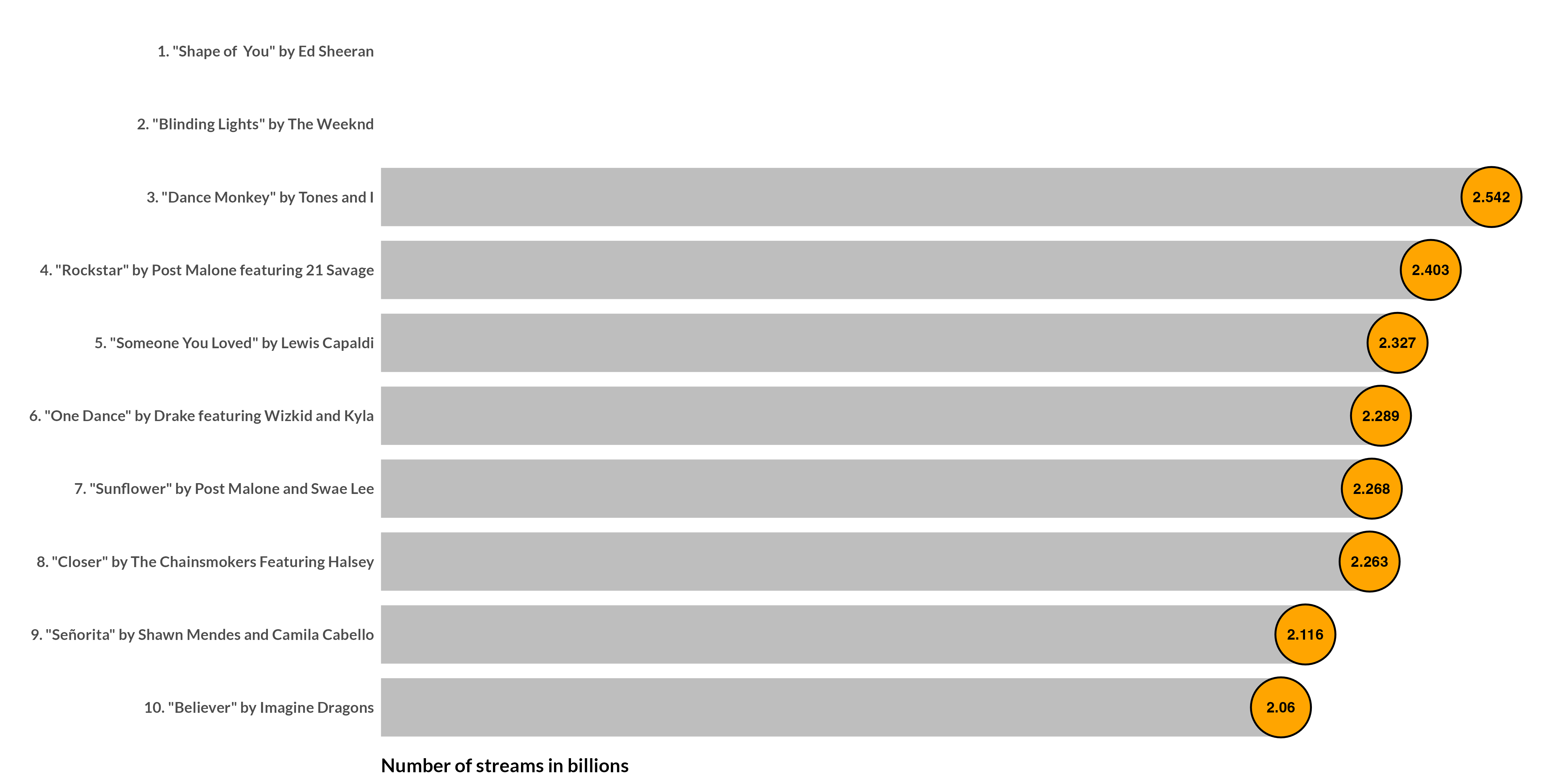### Citation

For attribution, please cite this work as

`Mock (2020, Aug. 1). The Mockup Blog: A bar chart 5 ways in ggplot2. Retrieved from https://themockup.blog/posts/2020-08-05-a-bar-chart-5-ways/`

BibTeX citation

```@misc{mock2020a,
author = {Mock, Thomas},
title = {The Mockup Blog: A bar chart 5 ways in ggplot2},
url = {https://themockup.blog/posts/2020-08-05-a-bar-chart-5-ways/},
year = {2020}
}```